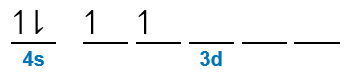# Problem: Give a possible set of values of the four quantum numbers for the 4s and 3d electrons in titanium.

🤓 Based on our data, we think this question is relevant for Professor Awino's class at ISU.

###### FREE Expert Solution

Titanium Electron Configuration:

Ti (atomic # = 22):       [Ar]4s23d2principal quantum number (n)  energy level in orbitals and its value could be any positive integer starting from 1 to infinity

angular momentum quantum number (ℓ) → (l) has to be at least 1 less than n, range of values from 0 up to (n-1)

▪ Each ℓ value corresponds to a subshell:

= 0 → s subshell
= 1 → p subshell
= 2 → d subshell
= 3 → f subshell

• magnetic quantum number (m) → range of values from - to +

▪ The m values (for each ℓ) represent an orbital for the subshell:

s subshell→ 1 orbital
p subshell→ 3 orbitals
d subshell → 5 orbitals
f subshell → 7 orbitals

spin quantum number (ms) → can only have two possible values +½ (spin up electron) or ½ (spin down electrons)

• Note that 1 orbital can hold a maximum of 2 electrons → 1 spin up (+½) and 1 spin down (–½)

For the 2s electrons:

4s → n = 4

▪ s subshell →  = 0

• for mrange of values from - to +

▪ since s subshell has only one orbital:    m → has only one value
m = 0

1 spin up electron → ms = +½
1 spin down electron → ms = –½

Possible Quantum Numbers:

n = 4            ℓ = 0               m = 0            ms = +½
n = 4            ℓ
= 0               m = 0            ms = –½

For the 3d electrons:###### Problem Details

Give a possible set of values of the four quantum numbers for the 4s and 3d electrons in titanium.